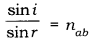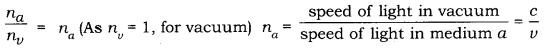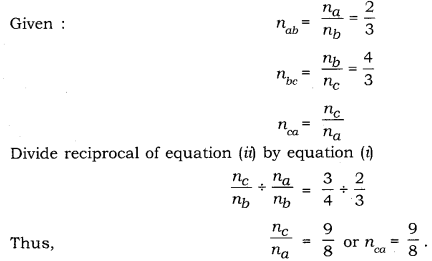# State Snell’s law of refraction of light

State Snell’s law of refraction of light. Write an expression to relate refractive index of a medium with speed of light in vacuum.
The refractive index of a medium ‘a’ with respect to medium ‘b’ is 2/3 and the refractive index of medium ‘b’ with respect to medium ‘c’ is 4/3. Find the refractive index of medium ‘c’ with respect to medium ‘a’.

Snell’s law of refraction: The ratio of the sine of the angle of incidence to the sine of the angle of refraction is constant. This is first law of refraction.
Mathematically, it can be given as follows:Here, nab is the relative refractive index of medium a (incident medium) with respect to medium b (refracted medium). If the light ray going from vacuum to medium a then the refractive index of medium a can be written as,where, c and v are the speeds of light in vacuum and medium a respectively.